# Clebsch condition

(diff) ← Older revision | Latest revision (diff) | Newer revision → (diff)

A necessary condition for optimality in the problem of variational calculus on a conditional extremum; established by R. Clebsch . Suppose an extremal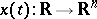provides a conditional minimum of the functional in the Bolza problem: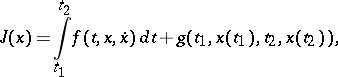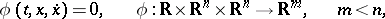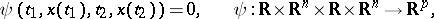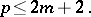Then, according to the multiplier method, it is an unconditional extremal of the functional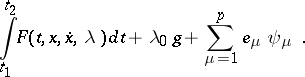(1)

Hereand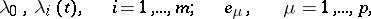are the Lagrange multipliers, which have to be determined together with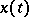from the necessary conditions for an extremum of the functional (1). One such necessary condition is the Clebsch condition.

In order thatbe minimal in the above problem, it is necessary that the Clebsch condition should hold. It requires that for any non-trivial set of numbers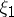,, satisfying the equations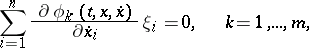the following quadratic form be non-negative:The Clebsch necessary condition is directly related to the stronger necessary Weierstrass conditions (for a variational extremum) and can be obtained as a corollary of the latter.

In problems of the calculus of variations on an unconditional extremum, in particular in the simplest problem of variational calculus, the analogue of the Clebsch condition is the Legendre condition.

In problems of optimal control, the Clebsch condition is equivalent to the non-positiveness of the second differential of the Hamilton function, which is a necessary condition in order that the Pontryagin maximum principle be fulfilled for optimal control in an open domain.

How to Cite This Entry:
Clebsch condition. Encyclopedia of Mathematics. URL: http://encyclopediaofmath.org/index.php?title=Clebsch_condition&oldid=16939
This article was adapted from an original article by I.B. Vapnyarskii (originator), which appeared in Encyclopedia of Mathematics - ISBN 1402006098. See original article